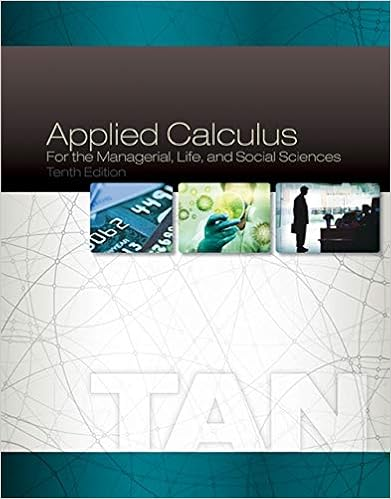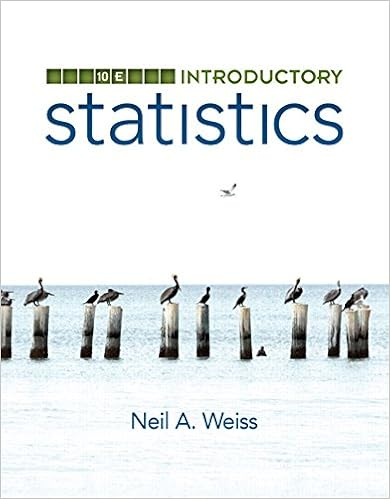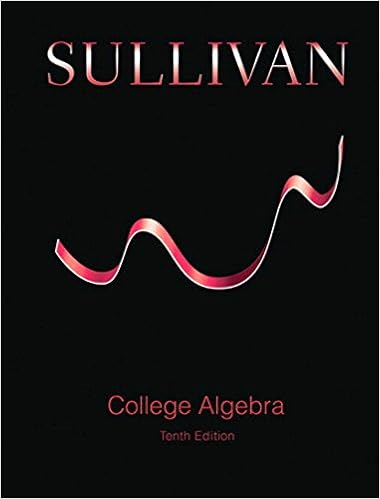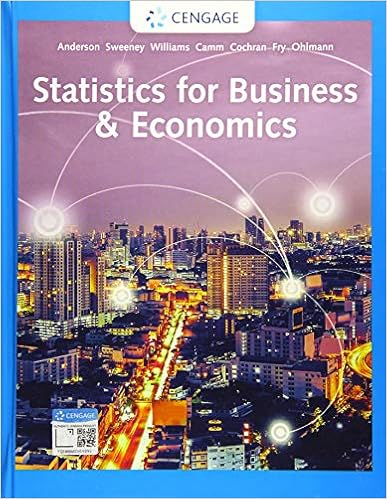# Differential Equations Exam Review (5 of 6) - Review 4:...

• Test Prep
• 5

This preview shows page 1 - 2 out of 5 pages.

The preview shows page 1 - 2 out of 5 pages.
Review 4: L22-L281. (1) Use variation of parameters to find( )1vtand( )2vt(up to a constant) that form aparticular solution to the nonhomogeneous equation, given two linear independentsolutions to the corresponding homogeneous equation. (2) Find and simplify (if possible)a particular solution,( )pyt. (3) Give the general solution to the nonhomogeneousequation.()25512515;51,tttytyyt eytye′′+===2. The equation and a non-trivial solution( )1ytto the equation are given. Find a secondlinearly independent solution.
13. A mass-spring system is governed by the differential equation30yy′′+=,( )01y=,( )01y= −The initial displacement here is 1 m to the right of the equilibrium position (positive) andthe initial velocity is 1 m/sec to the left (negative).(a) Determine the equation of the motion in terms of the sine function along withits amplitude, period, natural frequency, angular frequency, phase angle(πϕπ<), and phase shift,ϕω. Specify units for each of the quantities.(b) How long does it take after release to pass through the equilibrium position?(c) Graph the equation of the motion.4. Assume that the motion of a mass-spring system with damping is governed by2290d ydybydtdt++=;( )01y=,( )01y=ϕ.

Course Hero member to access this document

##### We have textbook solutions for you!The document you are viewing contains questions related to this textbook.
Chapter 5 / Exercise 1
Applied Calculus for the Managerial, Life, and Social Sciences
TanExpert VerifiedBrowse all Textbook Solutions

Course Hero member to access this document

End of preview. Want to read all 5 pages?

Course Hero member to access this document

Term
Fall
Professor
TUNCER
##### We have textbook solutions for you!
The document you are viewing contains questions related to this textbook.The document you are viewing contains questions related to this textbook.
Chapter 5 / Exercise 1
Applied Calculus for the Managerial, Life, and Social Sciences
TanExpert Verified
•••